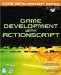# Generating Random Numbers

 [ LiB ]

### Generating Random Numbers

Believe it or not, random number generation can be very useful for creative results in a game. Random numbers are used to provide random screen locations for objects such as the stars in your star field. Another example of when you want random numbers to affect your game is when you want other game entities, such as enemies, to appear in random locations on the screen. Every time the game is played , they will always appear in a different location if random numbers are used to affect their position attributes. One reason for this is that the game player doesn't want your game to be predictable every time he plays. Even a game like Tetris generates a random block, out of 7 total blocks, until you lose the game. Games should be fun and the player should have a different adventure every time he plays (even if he stays at the same level).

NOTE

NOTE

Chapter 11 is full of useful game math, but if you would like further reading, check out other Game Development books published by Premier Press that cover the sub ject. Enjoy.

So, how do you generate random numbers? I'm not going to get into the complex math that it actually takes to generate them because doing so would take up at least a few chapters. Plus, this isn't a math book you're reading this to make games, not master mathematical functions.

Luckily, you don't have to reinvent the wheel. There is a Math object built into ActionScript that you can take full advantage of. I'm only going to touch upon a couple methods of the Math object. One method is:

` Math.random(); `

This method returns a number from 0 up to the number 1. If called upon twice, the second return will be completely unpredictable, just like the first returned value. Let's test it out.

` trace(Math.random()); `

I put this statement in a for loop that looped 20 times. This was my output:

` 0.96022649621591 0.798773859161884 0.999327154364437 0.441732861567289 0.526776063721627 0.939985535573214 0.126834238413721 0.12000940926373 0.931211666669697 0.688188867177814 0.779313802719116 0.476958607323468 0.5028145625256 0.957195661962032 0.958747376687825 0.932823874987662 0.665031484793872 0.0121707990765572 0.985493869520724 0.8782356120646 `

You could run the script a few times and see that this output would change every time. Zero is a very possible answer1 isn't. Keep that in mind. The number generated can get very close to 1and you can also use another method that rounds the result up or down. Either way, you can pretty much generate any possible range you want by multiplying random's result by any number.

To understand how this works, pay close attention to the following explanation. You know that when you multiply a number by 1, you get the number you multiplied by. If we multiply by .5, you get half of the number you multiplied by, and so on. So what would happen if we were to type in a line like this:

` trace(Math.random()*10); `

This would yield a number from 0 to a number very close to 10. In order for you to verify this, you would have to put the resulting number in a loop (just like in the last example). Go ahead and observe the output. I got the following:

` 7.51654958352447 6.19418734684587 8.9927756646648 9.64880893472582 7.58282233960927 0.215201745741069 5.46044206712395 1.21198146604002 2.19898341689259 1.69041742570698 1.39441115781665 9.54875849187374 4.76128031965345 6.64634562563151 8.05860636290163 0.770040606148541 1.14755514543504 4.76418022532016 8.93458025064319 0.0836741318926215 `

This is very coolyou can now generate a random set of numbers that are useful for all different genres of games. If you're dealing with an action game, you can use random numbers to decide when your enemy wants to fire explosives at you. If you're working on a puzzle game, you can easily use this to decide the next move that the computer opponent will make.

What if you aren't dealing with floating point numbers? What if you were only working with integers? How can you restrain your answers to only integers? It's easily done. After you scale the result from the random method, all you have to do is use the floor method on it. Just so you know, the floor method is specifically named the way it is because it rounds the floating-point value down to the nearest integer. Allow me to show you how this is done: trace(Math.floor(Math.random()*10));

Looks complicated, but it's not. All I did was wrap the floor method around the Math.random()*10 result. Type in the following loop in and see what it outputs:

` for (var index = 0; index < 20; index++)   trace(Math.floor(Math.random()*10)); `

Take a look at the output I got and observe how neat it is.

` 6 2 7 7 1 6 9 5  1 5 6 9 4 2 3 5 0 8 8 8 `

Cool, eh? Notice something else: I have results ranging from 0 to 9. What if you wanted results from 0 to 10? Even easier! All you have to do is add 1 to the result and you automatically push everything up one. I love game programmingdon't you?

 [ LiB ]Game Development with ActionScript
ISBN: 1592001106
EAN: 2147483647
Year: 2004
Pages: 162
Authors: Lewis Moronta